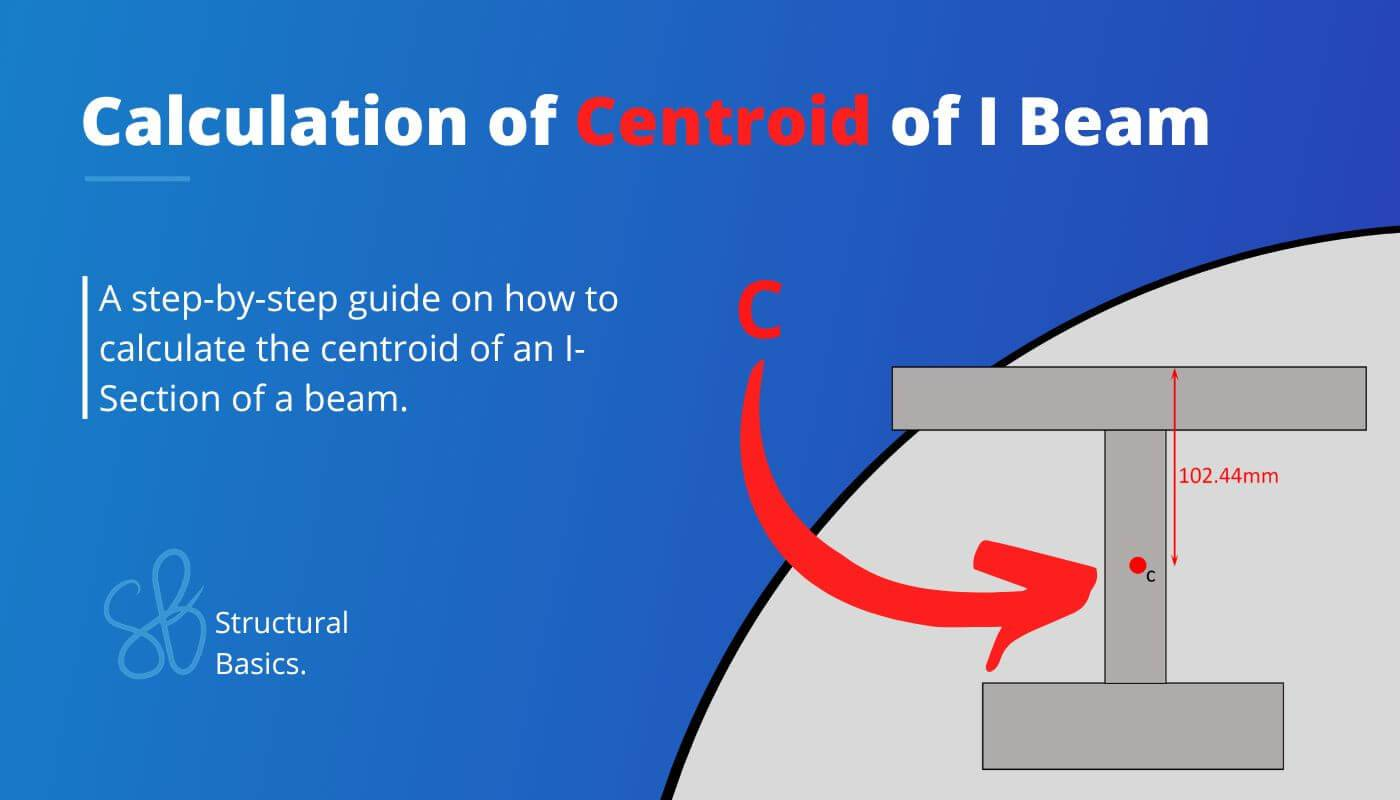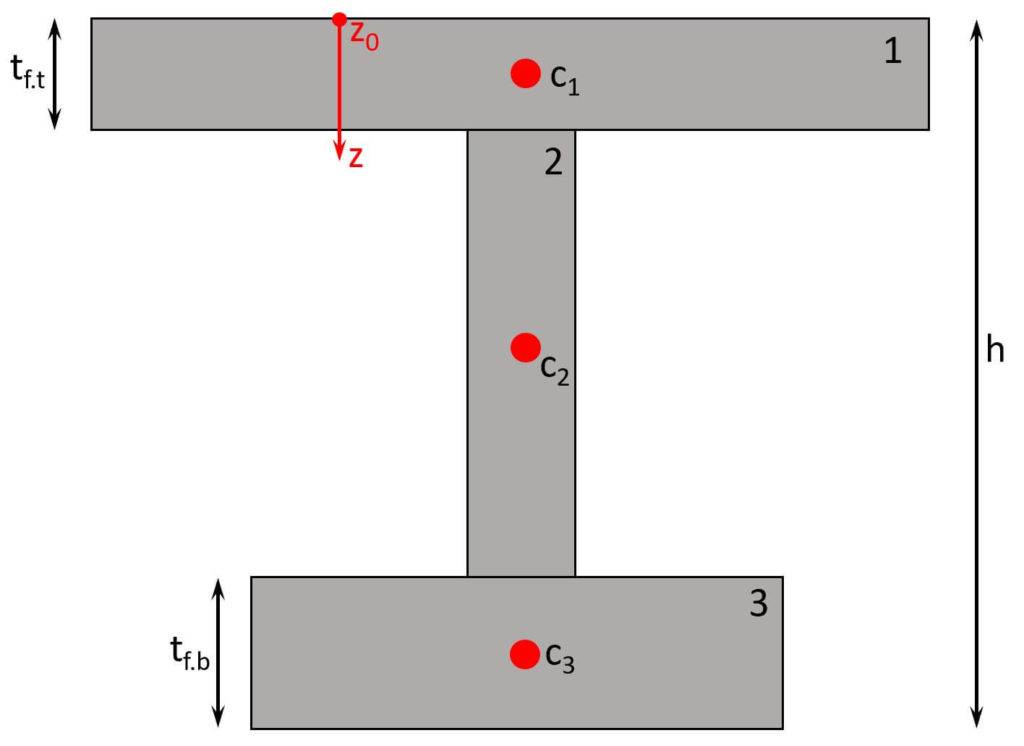# Centroid of I Beam: Calculation ExampleAre you an engineer, student, or someone who is looking to understand better how the centroid of an I Beam is calculated? 🙋‍♂️🙋‍♂️

The centroid of a section is a crucial parameter in calculating the Moment of inertia, which determines the strength of 3D objects such as beams, columns and slabs.

By understanding the calculation of the centroid, you are one step closer to designing an I- or H-Beam. 🔥🔥

In this beginner’s guide, we’ll walk you through the process of determining the centroid of an I Section, step-by-step.

Alright, let’s get started. 🚀🚀

## Procedure of Finding The Centroid of Any Section

There’s a simple step-by-step procedure that we can follow for any cross-section to calculate the centroid. 👍👍

Procedure:

1. Split the (Cross-) Section into its parts (see picture below). E.g. the I or H profile can be split into 3 parts, while the T-profile is split into 2 parts.
2. Find the centroid of each part.
3. With the centroid formula the centroid of the entire section (z-direction) is calculated:

$$z = \frac{\sum_{i=1}^{n} A_i \cdot z_i}{A}$$

With,

$A_i$ = area of a part i of the section
$z_i$ = vertical distance from reference point to the centroid of part i
$A$ = area of the sectionI-Section split up into 3 parts. Centroids of parts are displayed with red points (c1, c2, c3).

OK, now let’s apply this to the I Section. 👇👇

## Calculation of The Centroid of I Beam

Let’s calculate the centroid for the following example I Section.

Now, let’s also define the dimensions needed:

$t_{f.t}$ = 10mm
$t_{f.b}$ = 15mm
$h$ = 200mm
$w_{f.t}$ = 200mm
$w_{f.b}$ = 150mm
$t_w$ = 9mm

As we see from the formula above, we need to calculate

1. the area of each part i and
2. the centroid of each part i

So let’s do that. ⬇️⬇️

##### Area of part 1

$$A_1 = t_{f.t} \cdot w_{f.t} = 10mm \cdot 200mm = 2000mm^2$$

##### Area of part 2

$$A_2 = t_{w} \cdot (h-t_{f.t}-t_{t.b}) = 9mm \cdot (200mm-10mm-15mm) = 1575mm^2$$

##### Area of part 3

$$A_3 = t_{f.b} \cdot w_{f.b} = 15mm \cdot 150mm = 2250mm^2$$

##### Centroid of part 1

$$z_{c.1} = \frac{t_{f.t}}{2} = \frac{10mm}{2} = 5mm$$

##### Centroid of part 2

$$z_{c.2} = t_{f.t} + \frac{h-t_{f.t}-t_{t.b}}{2} = 10mm + \frac{200mm – 10mm – 15mm}{2} = 97.5mm$$

##### Centroid of part 3

$$z_{c.3} = h – t_{f.b} + \frac{t_{t.b}}{2} = 200mm – 15mm + \frac{15mm}{2} = 192.5mm$$

Alright, now let’s insert these results into the centroid formula

##### Centroid Formula

$$z = \frac{\sum_{i=1}^{n} A_i \cdot z_i}{A}$$
$$z = \frac{A_1 \cdot z_1 + A_2 \cdot z_2 + A_3 \cdot z_3}{A_1 + A_2 + A_3}$$
$$z = \frac{2000mm^2 \cdot 5mm + 1575mm^2 \cdot 97.5mm + 2250mm^2 \cdot 192.5mm}{2000mm^2 + 1575mm^2 + 2250mm^2}$$
$$z = 102.44mm$$

💡💡 Bonus Tip: If you need to calculate the centroid of a complex I Section or any Section in general, then you can use a Tool like Rhino where you draw the section. With a few simple steps you can then determine its centroid dimensions. 💡💡

Let us know in the comments below if you are interested in learning how to do it with Rhino. ✍️✍️

## What Is The Centroid of Section?

The centroid of a (cross-) section or profile is a point that represents the center of mass of a two-dimensional shape. It’s the point at which the entire area of the section can be assumed to be concentrated.

If a force is applied at that point, it will produce the same effect on the section as if the force were distributed uniformly across the entire area of the section.

The Centroid of Section is also used to calculate the Moment of Inertia and Section Modulus of cross-sections. These are 2 important properties used in structural design of elements such as beams, columns and slabs.

## Conclusion

Now, that you got an understanding of how to calculate the centroid of an i beam, you can learn about moment of inertia and cross-sectional area of i sections, because these parameters are based on the centroid of section.

If you want to learn where these cross-sectional properites are used in structural engineering, then check out the following articles.📖📖

I hope that this article helped you understand the centroid of section calculation and how to go further from here. In case you still have questions.

Let us know in the comments below. ✍️✍️